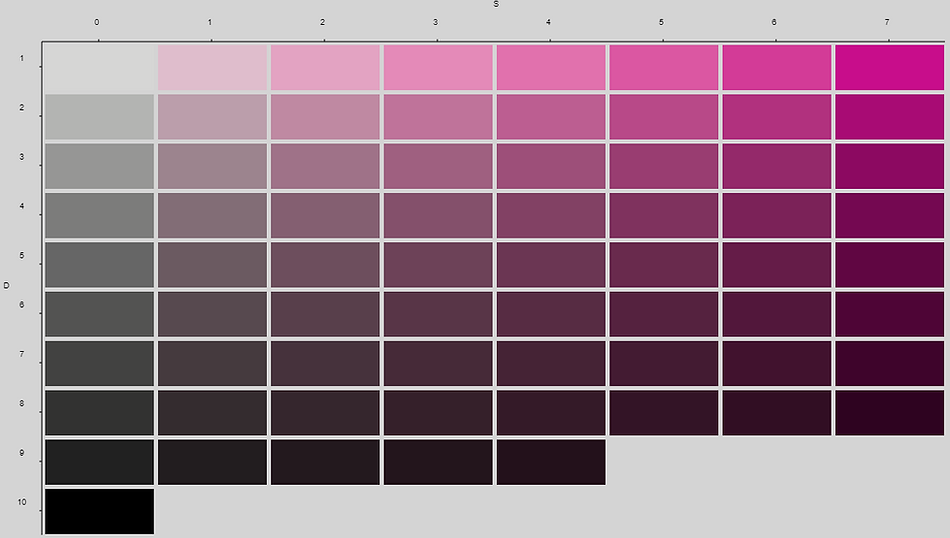top of page

# DIN 6164

In 1941 experiments began into determining a uniformly spaced hue circle by selecting 120 coloured samples from the Ostwald hue circle and asking subjects to arrange them in an orderly manner. The results were averaged and resulted in the determination of CIE chromaticity coordinates of points on 24 uniformly spaced hues.

The hues themselves are the lines starting from the neutral axis on the CIE 1931 chromaticity diagram (illuminant C), which pass through each of the individual points and terminate at the spectral curve or purple line. DIN hue is defined by constant dominant wavelength, which was known at the time not to conform with the perception of constant hue. The adoption of such a method for the construction of hues was a compromise between the requirements of perceptually equal spacing and ease of conversion between the DIN and CIE systems necessary for technical applications. DIN hue (DIN-Farbton) has the notation T and the 24 subdivisions form a closed circle beginning with T = 1 yellow, and progressing through reds, purples, blues and greens to T = 24 and back to T = 1. All possible hues can be expressed by decimal subdivisions of each of the individual hue steps.In 1945 experiments began to determine colours of equal saturation and lightness for each of the 24 basic hues. The colours with constant lightness and saturation formed a closed line of equal saturation around the hue circle: the saturation of this line was later defined as DIN saturation (DIN Sattigung) S = 6. The subdivision of the saturation scale along the constant hue line was achieved by equal visual scaling for only eight of the basic hues. The coordinates of these points were plotted on a u,v chromaticity diagram which displayed equal visual spacing on every hue line. The results were used to determine the coordinates of the subdivisions of the saturation axis for the remaining sixteen hues by interpolation and extrapolation along the constant hue lines. This is the second compromise between equality of visual spacing and ease of DIN to CIE conversion. The perceptually equal quality of the saturation steps is compromised by the advantage that for colorimetric description of hue and saturation, only the CIE coordinates of the 24 hues at saturation S = 6 are necessary to calculate all the hue and saturation lines. Additionally, it was assumed, and subsequently supported by Uchikawa, Uchikawa and Kaiser (1984), that the constant saturation lines are equivalent for all degrees of lightness.From Ostwald's work which emphasised that equally saturated colours of opposite hues are not psychologically equivalent when they have the same luminous reflectance. It was assumed, with reference to Rosch's (1928) and MacAdam's (1935) research, that this condition could be achieved by using equal relative luminance factors. The method for measuring lightness that was adopted for the DIN system is thus unique, and to reflect this the brightness coordinate was inverted and renamed DIN darkness (DIN Dunkelstufe), denoted by a D. The darkness axis is defined as the ratio of the luminance reflectance of the sample, Y, and the luminance reflectance of the optimal colour of equal chromaticity, Ao. Originally, colours of equal relative luminance were thought to be of equal brightness, however, this assumption could not later be verified. Research by Uchikawa, Uchikawa and Kaiser (1984) on comparisons of luminance - saturation dependence of equally bright colours has indicated that the slope is too steep, resulting in a drop in perceived brightness as saturation increases. Earlier research by Klughardt (1936) was used to determine the scaling of darkness along the achromatic axis, which was extended to encompass the chromatic colours by the inclusion of Ao. The darkness steps are defined by a modified Fechner formula, derived by Delboeuf (1873), as follows:

D = 10 - 6.1723log(40.7h + 1)

where h is constant relative lightness and equivalent to Y/Ao. The darkness scale extends from D = 0 (white) to D = 10 (black).The complete DIN notation to uniquely identify colours in DIN colour space is thus T:S:D. DIN hue (T) forms a closed circle with 1 <= T < 25 and T = 25 equivalent to T = 1. The saturation (S) axis extends from S = 0 for achromatic colours and increases along the constant hue line to an upper limit dependent on the extent of each hue line. The darkness (D) axis extends from absolute white, D = 0, to absolute black, D = 10, such that 0 <= D <= 10.

bottom of page